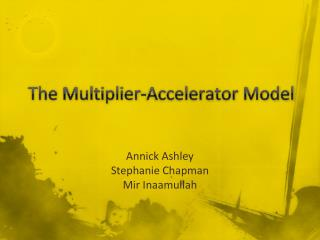# The Multiplier-Accelerator Model - PowerPoint PPT PresentationDownload PresentationThe Multiplier-Accelerator Model

The Multiplier-Accelerator ModelDownload Presentation## The Multiplier-Accelerator Model

- - - - - - - - - - - - - - - - - - - - - - - - - - - E N D - - - - - - - - - - - - - - - - - - - - - - - - - - -
##### Presentation Transcript

1. The Multiplier-Accelerator Model Annick Ashley Stephanie Chapman Mir Inaamullah

2. The Accelerator Effect • Measures how much the growth of the market economy alters the amount of private fixed investment • (i.e. investment in tangible capital goods or the replacement of depreciated ones). • Analyzing the Gross Domestic Product, (GDP) • As it increases businesses will, in theory, see rising profits, higher sales and more cash flow. • Business will then have more confidence in their ability to boost profit; thus they will more likely expend more money on private fixed investment.

3. The Multiplier Effect • Used more generally to measure how an exogenous variable affects an endogenous one. • Involves how one’s spending essentially becomes another’s income. • Ultimately, there is a much greater impact on the equilibrium of national income.

4. Aggregate Supply vs. Aggregate Demand • The vertical arrows represent a constant rise in demand • Horizontal arrows represent the resulting shift in supply from the two equilibrium points. Small change in the former creates a much greater difference in the latter. • Once aggregate demand rises expected sales and output increase higher employment rates and income more consumption raising aggregate demand yet again.

5. Example • Company invested 200 million dollars into a new manufacturing plant. • Expenditures. • The businesses supplying capital goods have new income • Then they, in theory, will spend around 3/5 of their new profits • Other businesses will gain \$120 million collectively. • Thus, the total economy has gained \$(200 + (3/5) * 200)) million • The producers who gained the \$120 million will likewise use 3/5 of their profits • We now have a total amount of \$(200 + ((3/5) * 200) + ((3/5) + 120)) of new income in the economy. • The cycle will continue but note that the rise in spending decreases by a fraction each step of the way.

6. Savings Rate • Investment into savings accounts can increase monetary circulation. • Federal Reserve sets the reserve requirement to 20% • A bank can loan up to \$80 of a 100-dollar customer deposit. • The \$80 will be, then, deposited into another bank that can then loan out another \$64 • It only truly affects circulation when money is deposited domestically, but it essentially gives the Fed great power to affect money supply. • If the Fed notes a recession in the economy and raises the reserve requirement to somewhere around 40%, much less money would be circulating in this same multiplier effect.

7. Building the Model • set up the model • estimate parameter values • v = capital stock ratio • s = savings rate • obtain final equation

8. Analyze the Bifurcation • origin goes from unstable to stable • must be a bifurcation! • super-critical Hopf s = 0.08 s = 0.10 s = 0.30

9. Application to Actual Data • check data over last 16 quarters • correlates with big drop • explanation: exogenous shock to the economy • RECESSION

10. Three-Dimensional Model • Model A • Model B **new parameter k: resistance of the savings rate to change

11. Conclusions • Multiplier-Accelerator model describes macro-economic trends • The two-dimensional model results in a Hopf bifurcation as the savings rate changes • The three-dimensional model results in periodic movement of the savings rate